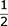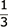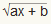### Site Tools

Illustrations

a:algebraic-characters

# Algebraic Characters

+ is the sign of addition; as c + d denotes that d is to be added to c;

- is the sign of subtraction; thus, c - d implies that d is to be subtracted from c.

x is the sign of multiplication; as c x d means the product of c and d.

÷ is the sign of division; c ÷ d signifies the quotient of c and d.

= is the sign of equality; thus c + d = e means the sum of c and d equals e.

√ is the sign of the square root; thus √x denotes the square root of a.

is the sign of the cube root, and generally any root of a quantity may be denoted by this sign, with the index of the root placed over it; thus signifies the cube root, the biquadrate root, etc.; but they may likewise be represented by the reciprocals of these indices ; as x, x, implying the square and cube roots of x.

A vinculum is a line drawn over several quantities, and means that they are taken together, assignifies the square root of ax and b. — Phillips's Compendium of Algebra. 12mo. 1824.

### Page Tools